# Predictive Soave-Redlich-Kwong (PSRK)

### Generic cubic equation of state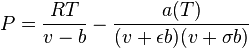$P = { { R T } \over { v - b } } - { { a(T) } \over { ( v + \epsilon b ) ( v + \sigma b ) } }$

Parameters for Soave-Redlich-Kwong equation are :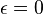$\epsilon = 0$ and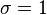$\sigma = 1$

Thus: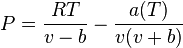$P = { { R T } \over { v - b } } - { { a(T) } \over { v ( v + b ) } }$

### PSRK mixing rule for calculating a(T) and b

Cohesion pressure (attractive parameter)  :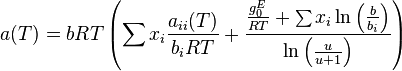$a(T) = b RT \left( \sum x_i { {a_{ii}(T)} \over {b_i R T} } + { \frac{ { \frac{g_0^E}{R T} } + \sum x_i \ln \left( \frac{b}{b_i} \right) }{ \ln \left( \frac{u}{u + 1} \right)} } \right)$ at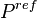$P^{ref}$ = 1 atm

with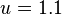$u = 1.1$,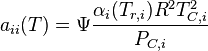$a_{ii}(T) = \Psi \frac{ \alpha_i (T_{r,i}) R^2 T_{C,i}^2 }{ P_{C,i} }$, and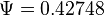$\Psi = 0.42748$.

Excluded volume or "co-volume" (repulsive parameter):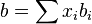$b = \sum x_i b_i$

where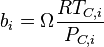$b_i = \Omega { \frac{ RT_{C,i} }{ P_{C,i} } }$, and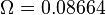$\Omega = 0.08664$

### Mathias-Copeman equation

Fitting experimental data with Mathias-Copeman parameters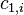$c_{1,i}$,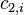$c_{2,i}$ and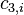$c_{3,i}$: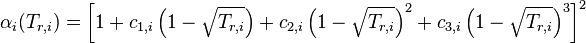$\alpha_i (T_{r,i}) = \left[ 1 + c_{1,i} \left(1 - \sqrt{T_{r,i}} \right) + c_{2,i} \left(1 - \sqrt{T_{r,i}} \right)^2 + c_{3,i} \left(1 - \sqrt{T_{r,i}} \right)^3 \right]^2$

General form if no experimental data available: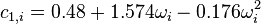$c_{1,i} = 0.48 + 1.574 \omega_i - 0.176 \omega_i^2$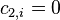$c_{2,i} = 0$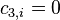$c_{3,i} = 0$

### Gibbs-Excess energy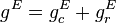$g^E = g_{c}^E + g_{r}^E$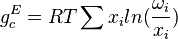$g_{c}^E = RT \sum x_i ln( {{\omega_i} \over {x_i}} )$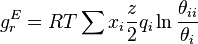$g_{r}^E = RT \sum x_i \frac{z}{2} q_i \ln \frac{\theta_{ii}}{\theta_i}$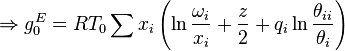$\Rightarrow g_{0}^E = R T_0 \sum x_i \left( \ln \frac{\omega_i}{x_i} + \frac{z}{2} + q_i \ln \frac{\theta_{ii}}{\theta_i} \right)$

### UNIFAC

Molecular volume parameter for component i (k: subgroup index) :$r_i = \sum_k \nu_k^{(i)} R_k$

Molecular surface area parameter for component i (k: subgroup index):$q_i = \sum_k \nu_k^{(i)} Q_k$

where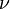$\nu$ is the number of the particular subgroups which a component i can be divided into.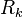$R_k$ is the volume parameter and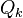$Q_k$ the surface area parameter for subgroup k.$R_k$ and$Q_k$ are tabulated parameters and provided by the UNIFAC Consortium (http://unifac.ddbst.de/). Each subgroup can be assigned to a main group.

Modified volume fraction [Kikic et al.; 1980]: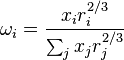$\omega_i = {{x_i r_i^{2/3}} \over {\sum_j x_j r_j^{2/3}}}$

Group mole fraction of subgroup k in component i :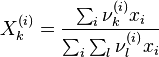$X_k^{(i)} = {{\sum_i \nu_k^{(i)} x_i} \over {\sum_i \sum_l \nu_l^{(i)} x_i}}$

Surface area fraction of subgroup k in component i: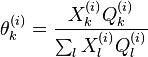$\theta_k^{(i)} = \frac{X_k^{(i)} Q_k^{(i)}}{\sum_l X_l^{(i)} Q_l^{(i)}}$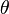$\theta$ is a matrix where the columns make up the components in the mixture and the rows are made up by the subgroups.

Local surface area fraction for j around i (the dot-product is performed of every single subgroup-row of matrix$\theta$ with the columns of matrix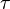$\tau$):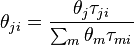$\theta_{ji} = { {\theta_j \tau_{ji}} \over {\sum_m \theta_m \tau_{mi}} }$

where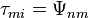$\tau_{mi} = \Psi_{nm}$

and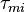$\tau_{mi}$ is the Boltzmann factor and can be calculated by transposing the main group interaction parameter matrix:$\Psi_{nm} = exp(- { {a_{nm} + b_{nm} T + c_{nm} T^2} \over {T} })$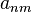$a_{nm}$,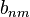$b_{nm}$ and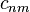$c_{nm}$ are the binary interaction parameters representing the interaction between the main groups where the following applies: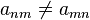$a_{nm} \ne a_{mn}$;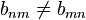$b_{nm} \ne b_{mn}$;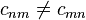$c_{nm} \ne c_{mn}$

Interaction parameters between identical main groups become 0.

The indexes n and m refer to subgroups. Thus, parameters a,b and c of different subgroups belonging to the same main group are identical. A subgroup to maingroup lookup has to be made when generating the data matrices for a, b and c.

The binary interaction parameters are also tabulated parameters and provided by the UNIFAC Consortium (http://unifac.ddbst.de/). The original UNIFAC model uses only$a_{nm} \ne a_{mn}$ as interaction parameters. Modified UNIFAC and PSRK include$b_{nm} \ne b_{mn}$ and$c_{nm} \ne c_{mn}$ for describing main group interactions.

A Excel file written by Carl Lira (http://www.egr.msu.edu/~lira/) can help to understand UNIFAC calculations. Take a look at the ACTCOEFF.XLS file under http://www.egr.msu.edu/~lira/computer/EXCEL/.

The UNIFAC consortium has published all parameters for the original UNIFAC model: http://www.ddbst.com/published-parameters-unifac.html

For obtaining the parameters for applying the modified UNIFAC (Dortmund) model or the PSRK model one has to become a member of the UNIFAC consortium or has to have access to the appendix of the following paper: doi:10.1016/j.fluid.2004.11.002

These pages can also help to understand this topic:

### Procedure for calculating vapor-liquid equilibria (VLE) (phi-phi approach)

Equilibrium condition :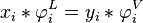$x_i * \varphi_i^L = y_i * \varphi_i^V$

Fugacity coefficient of the PSRK equation for component i in a mixture: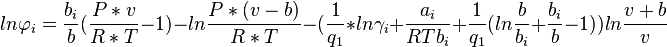$ln \varphi_i = \frac{b_i}{b} (\frac{P*v}{R*T} - 1) - ln \frac{P*(v-b)}{R*T} - ( \frac{1}{q_1} * ln \gamma_i + \frac{a_i}{RTb_i} + \frac{1}{q_1}(ln \frac{b}{b_i} + \frac{b_i}{b} - 1)) ln \frac{v+b}{v}$

with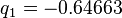$q_1 = -0.64663$

K-factor: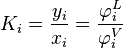$K_i = { {y_i} \over {x_i} } = { {\varphi_i^L} \over {\varphi_i^V} }$

Sum of mole fractions: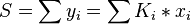$S = \sum y_i = \sum K_i * x_i$

Flow diagram for calculating isothermal VLE using PSRK: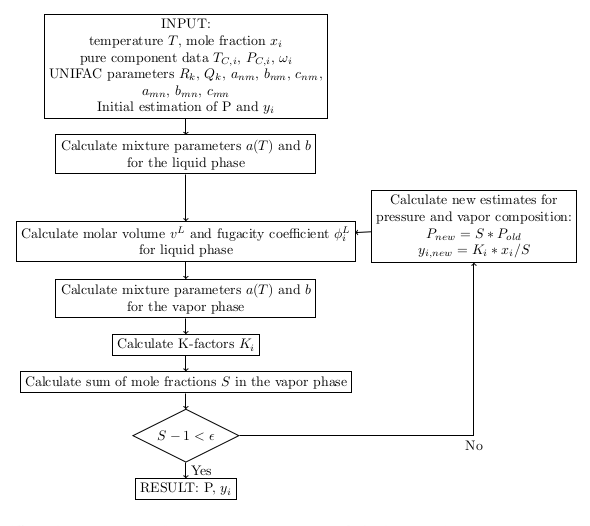Flow diagram of the phi-phi approach for calculating isothermal vapor-liquid equilibria (VLE) with the predictive Soave-Redlich-Kwong equation of state (EOS) File:Phi-phi approach PSRK.pdf